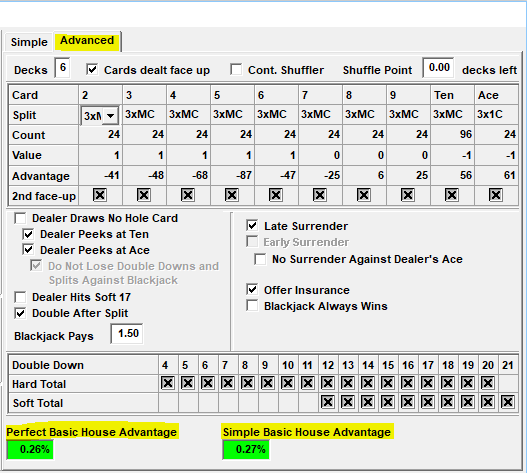Search
•Oliver

# Not so basic: Basic Strategy

Anybody who has looked at the details of BJSurvey's Sequence or Decision Reports probably noticed that the Basic Strategy used by BJSurvey is slightly different from published charts - and there are two good reasons for that.

First, Basic Strategy changes depending on the rules. You can display the Basic Strategy chart for a specific rule set from the Rule window.Second, almost all published charts use Simplified Basic Strategy, where BJSurvey uses Perfect Basic Strategy (a.k.a. Compositional Basic Strategy). Simplified Basic Strategy only takes the player’s total into account (e.g. 15) while Perfect Basic Strategy uses the composition of the player's hand (e.g. 5+10, 6+9, 7+8). The above mentioned charts will show that, but only for the first two cards of the player. BJSurvey uses that method beyond two cards (e.g. 5+3+7, etc...).Depending on the composition of the player's cards a 15 vs Ten calls for a Hit or Surrender.A 6+9 against a Ten calls for Surrender, while a 7+8 calls for a Hit.

Most players use Simplified Basic Strategy. So why does BJSurvey use Perfect Basic Strategy? Perfect Basic Strategy is also used when the house advantage for a specific rule set is calculated. Therefore, BJSurvey has to use Perfect Basic Strategy for maximum accuracy when calculating "Total Casino Advantage".The "Advanced" tab of the Rule window shows the House Advantage of Perfect and Simple Basic Strategy.